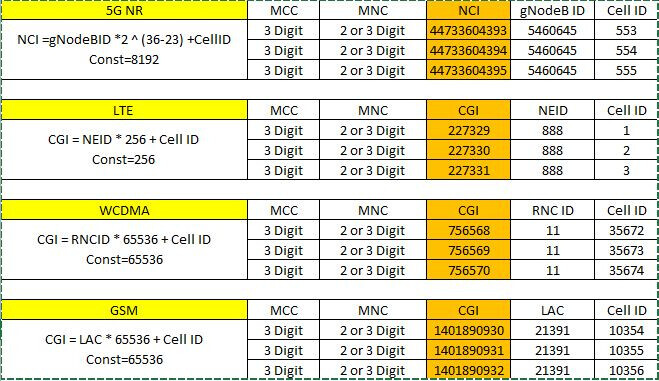# What is the formula for Cell ID (ECI) in LTE networks?

When using GMON in a LTE (4G) Network, i see a cell identification (ECI) with a very large number.

Can anyone tell me how do I calculate this ID?

BR,
Rafael

Admin note: this post was updated with image below.4 Likes

Hello @rafaeldamasio

The formula is: ECI = 256 * eNBId + Cellid

For Huawei, you can use ECI: 256 * [ENodeBFunction].[eNBId] + [EUtranCellFDD].[Cellid]
And for Ericsson ECI = 256 * [eNodeB].[ENODEBID] + [Cell].[Cellid]

BR
Carlos Alberto

11 Likes

@Carlos_Alberto can you explain further on why number 256, is it the data type UINT8 thats used to describe the same ?

I am not an expert But

256 = 100 in Hex so adding bit to get 28 for ECI

1 Like

From lncel export you can find the eci directly…

1 Like

Thanks a lot! Very useful.

1 Like

Dear All

I have ECI value 0x09DD748 , Could you kindly explain how do i derive actual cell id form this value, this is a HUAWEI EPC CDR record (user location information tag)

Hi dears,
Please, to calculate EUTRANCELLID, I apply:
(256 * enodBid) + sector ID
or
(256 * enodBid)+ LCRID?
Vendor ZTE.

1 Like

Generaly it’s lnbtsid * 256 + lcrid.

1 Like

Hi,

Cellid is the first two least significant hex digits (8binary digits) in the GCI.

So to add it to enbid you have to shift the enbid by these two hex digit and leave the two least significant digits to the cellid.

you make this shift by multiplying the enbid by 2^8=256, then you sum the cellid to the result.

it is like when you want to move from the ones to the tens then to hundreds then to thousands …etc. You multiply by 10 in each step. In our case, you multiply by 2 in each step; you need eight steps so it is 2^8=265

1 Like

Hello dears… any change in calculation for 5G NR? What is the formula for Cell ID (NCI) in 5G NR networks?

Hi Experts.
In LTE, we calculate the ECGI as 256 x ENB ID ( Normally 20 bits ) + Cell ID ( 8 bits).
So my question is: why we are multiplying with 256?

1 Like

It helps to convert 8 bit no to 16 bit no, for NR also same method.

8 bit no to 16 bit no means?
Which 8 bit no?

for conversion of Hexa Decimal need to multiply by 256

Lets say you have ECGI as 1035474,

ENODEB ID EXTRACTION:
=DEC2HEX(1035474,7) = 00FCCD2 >> To convert to Hex, If already hex this step is not needed,
=LEFT(00FCCD2 , 5) = 00FCC >> Take the left 5 digits
=HEX2DEC(00FCC) = 4044 >> convert to decimal

CELL ID EXTRACTION:
=RIGHT(00FCCD2 , 2) = D2 >> Take the rightmost 2 digits
=HEX2DEC(D2) = 210 >> convert to decimal

So the enodeb id is =HEX2DEC(LEFT(A3, 5)) = 40407
Cell id is =HEX2DEC(RIGHT(A3, 2)) = 72